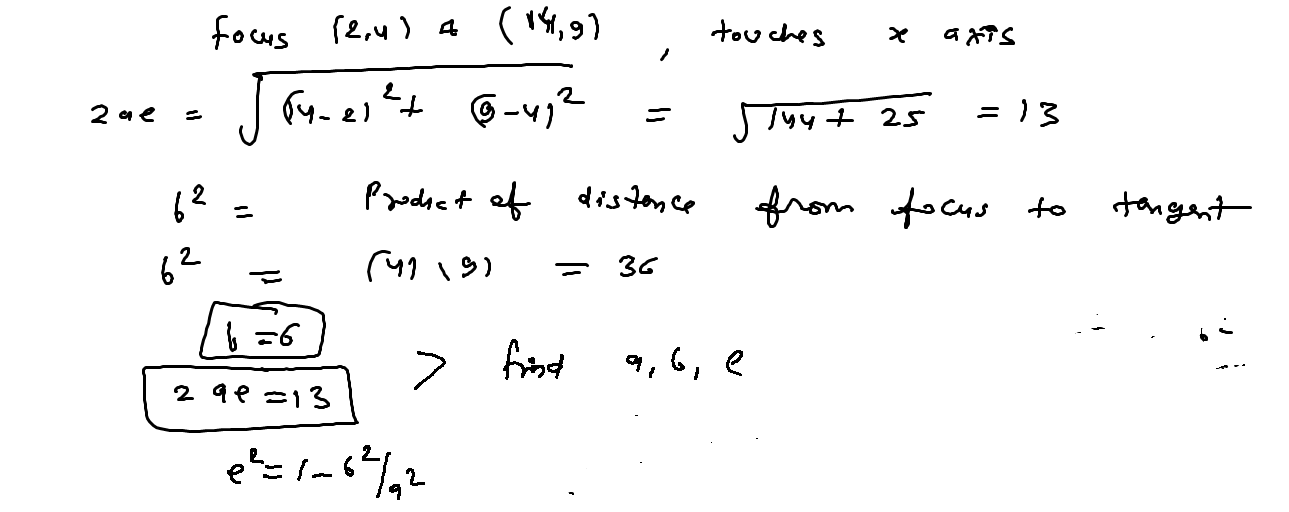Click to Chat

1800-1023-196

+91-120-4616500

CART 0

• 0

MY CART (5)

Use Coupon: CART20 and get 20% off on all online Study Material

ITEM
DETAILS
MRP
DISCOUNT
FINAL PRICE
Total Price: Rs.

There are no items in this cart.
Continue Shopping
`        Find eccentricities whose foci 2,4 and14,9 touches x-axis`
one year agoDeepak Kumar Shringi
4399 Points
``````
one year ago
```							 since ellipse touches the x-axis, therefore x-axis is a tangent to the ellipse. The product of the perpendicular distance is equal to square of the semi minor.Therefore b2=9*4=36distance between focii = 2ae=13ae=13/2a2-b2=169/4a2=169/4 + 36 =313/4e= square root of( a2-b2/a2)=square root of(313/36) RegardsArun  since ellipse touches the x-axis, therefore x-axis is a tangent to the ellipse. The product of the perpendicular distance is equal to square of the semi minor.Therefore b2=9*4=36distance between focii = 2ae=13ae=13/2a2-b2=169/4a2=169/4 + 36 =313/4e= square root of( a2-b2/a2)=square root of(313/36)
```
one year ago
Think You Can Provide A Better Answer ?

## Other Related Questions on Analytical Geometry

View all Questions »### Course Features

• 731 Video Lectures
• Revision Notes
• Previous Year Papers
• Mind Map
• Study Planner
• NCERT Solutions
• Discussion Forum
• Test paper with Video Solution### Course Features

• 53 Video Lectures
• Revision Notes
• Test paper with Video Solution
• Mind Map
• Study Planner
• NCERT Solutions
• Discussion Forum
• Previous Year Exam Questions